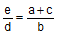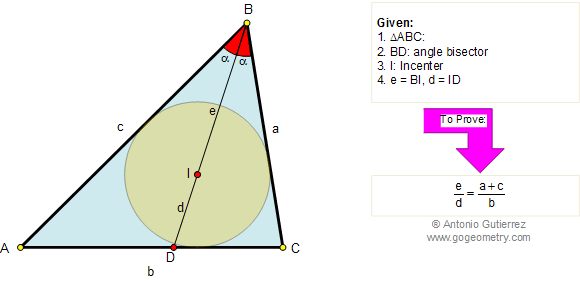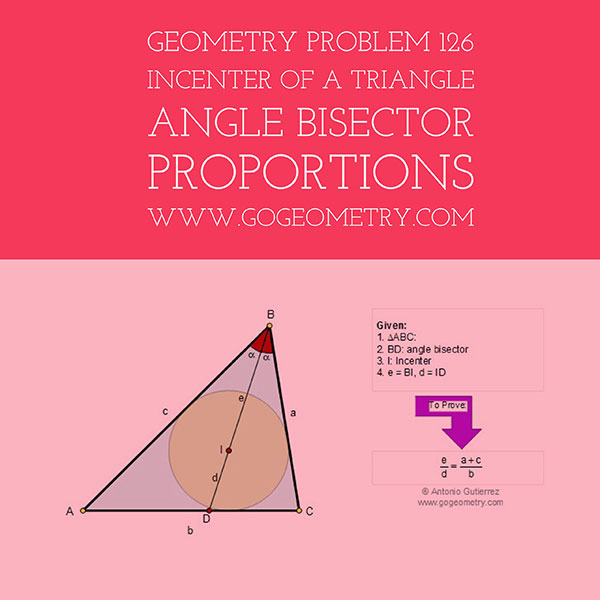Problem 126. Incenter of a Triangle, Angle Bisector, Proportions. Level: High School, College, SAT Prep. In the figure below, given a triangle ABC of sides AB = c, BC = a, and AC = b. Let be BD the angle bisector of B, I the incenter, BI = e and ID =d. Prove that:. View or post a solution.Illustration using iPad Apps.See: Euclid's Elements Book VI, Proposition 3: Angle Bisector Theorem. Recent Additions